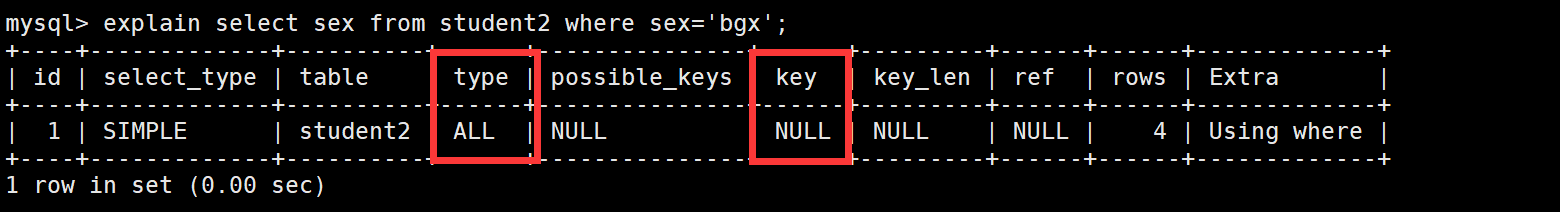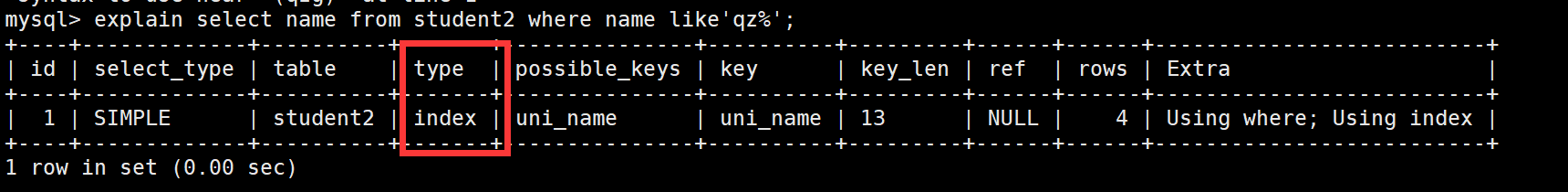•闪存
•博客
• 发言小组
• 投递新闻
• 提问博问
• 添加收藏
• 发布招聘
•文库

# 关于sql优化的问题

0悬赏园豆：50 [待解决问题]SELECT DISTINCT
Id ,
Number ,
Name ,
Time
FROM ( SELECT
Id ,
Number ,
Name ,
Time
FROM table_a a
WHERE Time = ( SELECT MAX(Time)
FROM table_a b
WHERE a.Id = b.Id
)
UNION ALL
SELECT
Id ,
Number ,
Name ,
Time
FROM table_b a
WHERE Time = ( SELECT MAX(Time)
FROM table_b b
WHERE a.Id = b.Id
)
) a

0

``````SELECT DISTINCT
Id,
Number,
NAME,
Time
FROM
(
SELECT
Id,
Number,
NAME,
Time
FROM
table_a a
WHERE
Time = (
SELECT
MAX(Time)
FROM
table_a b
WHERE
a.Id = b.Id
)
UNION ALL
SELECT
Id,
Number,
NAME,
Time
FROM
table_b a
WHERE
Time = (
SELECT
MAX(Time)
FROM
table_b b
WHERE
a.Id = b.Id
)
) a``````

0

Time =() 尝试修改为 not exists (..a.time<b.time..)

0

1）index / all
2）range
3）ref
4）eq_ref
5）const
6）system
7）null0

SiPenglei | 园豆：118 (初学一级) | 2019-03-26 22:44
0

SELECT Id ,Number ,Name ,Time FROM table_a a WHERE Time = (

SELECT MAX(Time) FROM table_a b WHERE a.Id = b.Id )

UNION

SELECT Id ,Number ,Name ,Time FROM table_b a WHERE Time = (

SELECT MAX(Time) FROM table_b b WHERE a.Id = b.Id )

AYard | 园豆：436 (菜鸟二级) | 2019-03-27 10:04

@李小小丶: 你这两个子句是不是写错了,它的结果和这句是一样的

"SELECT Id ,Number ,Name ,Time FROM table_a a WHERE Time = (

SELECT Time FROM table_a b WHERE a.Id = b.Id ) "

"SELECT Id ,Number ,Name ,Time FROM table_a WHERE Time = (

SELECT MAX(Time) FROM table_a ) "

0

yanch | 园豆：204 (菜鸟二级) | 2019-03-28 19:04

您需要登录以后才能回答，未注册用户请先注册